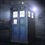# PHP practice for RMO #1

Let $n \geq 3$ be an odd number.Show that there is a number in the set,$\{2^1-1,2^2-1,...2^{n-1}-1\}$,which is divisible by $n$.5 years, 8 months ago

This discussion board is a place to discuss our Daily Challenges and the math and science related to those challenges. Explanations are more than just a solution — they should explain the steps and thinking strategies that you used to obtain the solution. Comments should further the discussion of math and science.

When posting on Brilliant:

• Use the emojis to react to an explanation, whether you're congratulating a job well done , or just really confused .
• Ask specific questions about the challenge or the steps in somebody's explanation. Well-posed questions can add a lot to the discussion, but posting "I don't understand!" doesn't help anyone.
• Try to contribute something new to the discussion, whether it is an extension, generalization or other idea related to the challenge.

MarkdownAppears as
*italics* or _italics_ italics
**bold** or __bold__ bold
- bulleted- list
• bulleted
• list
1. numbered2. list
1. numbered
2. list
Note: you must add a full line of space before and after lists for them to show up correctly
paragraph 1paragraph 2

paragraph 1

paragraph 2

[example link](https://brilliant.org)example link
> This is a quote
This is a quote
    # I indented these lines
# 4 spaces, and now they show
# up as a code block.

print "hello world"
# I indented these lines
# 4 spaces, and now they show
# up as a code block.

print "hello world"
MathAppears as
Remember to wrap math in $$ ... $$ or $ ... $ to ensure proper formatting.
2 \times 3 $2 \times 3$
2^{34} $2^{34}$
a_{i-1} $a_{i-1}$
\frac{2}{3} $\frac{2}{3}$
\sqrt{2} $\sqrt{2}$
\sum_{i=1}^3 $\sum_{i=1}^3$
\sin \theta $\sin \theta$
\boxed{123} $\boxed{123}$

Sort by:

The question is easy. My proof: If say some of the numbers is divisible by n then we are done. If no then there must be two numbers which must be congruent to the same number x(mod n). Let those two numbers be (2^i-1) and (2^j-1). Then subtract the smaller from the larger and factorize. WLOG let i>j. Then u get 2^j common outside and inside the bracket u get (2^(i-j)-1). Now since n is odd, gcd(n,2^j)=1. So n divides (2^(i-j)-1) and note that this quantity lies in the set and hence you are done :)

Sorry for not using Latex as I am in a hurry right now !

- 5 years, 8 months ago

That is a nice solution and no problem!

- 5 years, 8 months ago

If I can ask,are you a RMO participant?

- 5 years, 8 months ago

Yes

- 5 years, 8 months ago

This year?

- 5 years, 8 months ago

Yes

- 5 years, 8 months ago

Which class?

- 5 years, 8 months ago

This is my last year I am in 11th

- 5 years, 8 months ago

Ohh Well,best of luck!

- 5 years, 8 months ago

Seems like I have got a tough competitor from my state ;)

- 5 years, 8 months ago